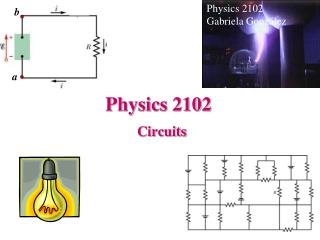DownloadDownload PresentationPhysics 2102

# Physics 2102

Download Presentation## Physics 2102

- - - - - - - - - - - - - - - - - - - - - - - - - - - E N D - - - - - - - - - - - - - - - - - - - - - - - - - - -
##### Presentation Transcript

1. Physics 2102 Gabriela González b a Physics 2102 Circuits

2. Recall:Resistors and Capacitors ResistorsCapacitors Key formula: V=iR Q=CV In series: same current same charge Req=∑Rj 1/Ceq=∑1/Cj In parallel: same voltage same voltage 1/Req=∑1/Rj Ceq=∑Cj

3. Recall: DC circuits • Loop rule: when walking along a loop, add potential differences across each element, and make the total equal to zero when you come back to the original point. • Junction rule: at every junction, total current is conserved. • Problem strategy: • Replace resistors in series and in parallel with their equivalent resistors • Draw currents in every wire, and label them • Write the loop rule for each loop (or for the loop that involves your question) • Write the junction rules for the currents. • Solve the equations for the currents. • Answer the question that was asked.

4. Example • Which circuit has the largest equivalent resistance? • Assuming that all resistors are the same, which one dissipates more power? • Which resistor has the smallest potential difference across it?

5. Assume the batteries are ideal, and have emfE1=12V, E2=9V, and R=3W. Which way will the current flow? Which battery us doing positive work? If the potential at A is 0V, what is the potential at B? How much power is dissipated by the resistor? How much power is delivered (or absorbed) by the batteries? Example

6. Example Find the equivalent resistance between points (a) F and H and (b) F and G. (Hint: For each pair of points, imagine that a battery is connected across the pair.)

7. Light bulbs • If all batteries are ideal, and all batteries and lightbulbs are identical, in which arrangements will the lightbulbs as bright as the one in circuit X?

8. i(t) E/R RC circuits: charging a capacitor In these circuits, current will change for a while, and then stay constant. We want to solve for current as a function of time i(t). The charge on the capacitor will also be a function of time: q(t). The voltage across the resistor and the capacitor also change with time. To charge the capacitor, close the switch on a. E + VR(t)+VC(t) =0 E -i(t)R -q(t)/C = 0 E - (dq(t)/dt) R - q(t)/C =0 A differential equation for q(t)! The solution is: q(t) = CE(1-e-t/RC) And then i(t) = dq/dt= (E/R) e-t/RC Time constant=RC

9. i(t) + C E/R - RC circuits: discharging a capacitor Assume the switch has been closed on a for a long time: the capacitor will be charged with Q=CE. +++ --- Then, close the switch on b: charges find their way across the circuit, establishing a current. VR+VC=0 -i(t)R+q(t)/C=0 => (dq/dt)R+q(t)/C=0 Solution: q(t)=q0e-t/RC=CEe-t/RC i(t) = dq/dt = (q0/RC) e-t/RC = (E/R) e-t/RC

10. Example The three circuits below are connected to the same ideal battery with emf E. All resistors have resistance R, and all capacitors have capacitance C. • Which capacitor takes the longest in getting charged? • Which capacitor ends up with the largest charge? • What’s the final current delivered by each battery? • What happens when we disconnect the battery?

11. Example In the figure, E= 1 kV, C = 10 µF, R1 = R2 = R3 = 1 MW. With C completely uncharged, switch S is suddenly closed (at t = 0). • What’s the current through each resistor at t=0? • What’s the current through each resistor after a long time? • How long is a long time?